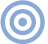# Reference

## The Essex Echo 1995: Electrical Signal Propagation & Cable Theory Maxwell's Equations

Sidebar 2: Maxwell's Equations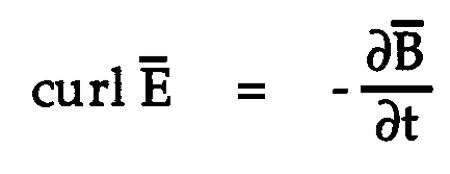Ampere's Law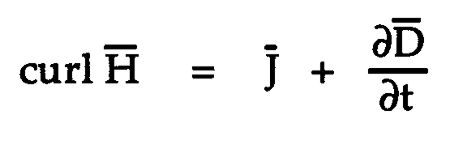Gauss's Theorem

div D-Bar = r

No Magnetic Monopoles

div B-Bar = 0

The constituent relationships that define electrical and magnetic material properties are

D-Bar = ε0εrE-Bar
B-Bar = µ0µrH-Bar
J-Bar = σE-Bar (if σ is constant, then this equation represents Ohm's Law)

However, it is common to write ε = ε0εr and µ = µ0µr, thus D-Bar = εE-Bar and B-Bar = µH-Bar
where
E-Bar, electric field strength, (volt/m)
B-Bar, magnetic flux density, (tesla)
D-Bar, electric flux density, (coulomb/m2)
r, charge density, (coulomb/m3)
σ, conductivity, (ohm-m)–1 (conductivity is the reciprocal of resistivity)
H-Bar, magnetic intensity, (ampere-(turn)-m)
J-Bar, current density, (ampere/m2)
ε0, permittivity of free space, (farad/m)
εr, relative permittivity
µ0, permeability of free space, (henry/m)
µr, relative permeability
t, time, (second)

Editor's Note: The bar over some parameters in the equations indicates vector or directed quantities. These are also represented in the text in word form, ie, H-Bar.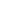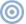ARTICLE CONTENTSX